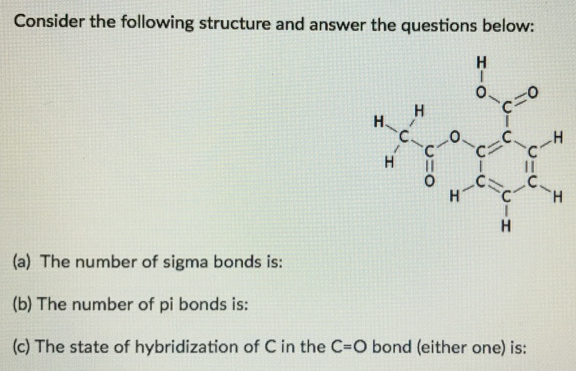# Problem: Consider the following structure and answer the questions below:a. The number of sigma bonds is:b. The number of pi bonds is:c. The state of hybridization of C in the C=O bond (either one) is:

###### FREE Expert Solution
100% (217 ratings)###### Problem Details

Consider the following structure and answer the questions below:

a. The number of sigma bonds is:

b. The number of pi bonds is:

c. The state of hybridization of C in the C=O bond (either one) is:Frequently Asked Questions

What scientific concept do you need to know in order to solve this problem?

Our tutors have indicated that to solve this problem you will need to apply the Hybridization concept. You can view video lessons to learn Hybridization. Or if you need more Hybridization practice, you can also practice Hybridization practice problems.